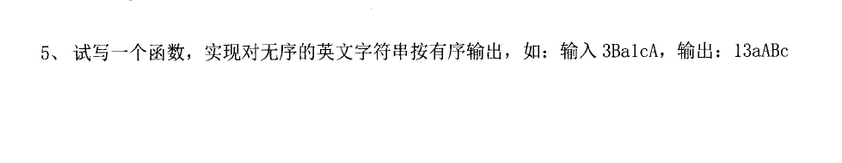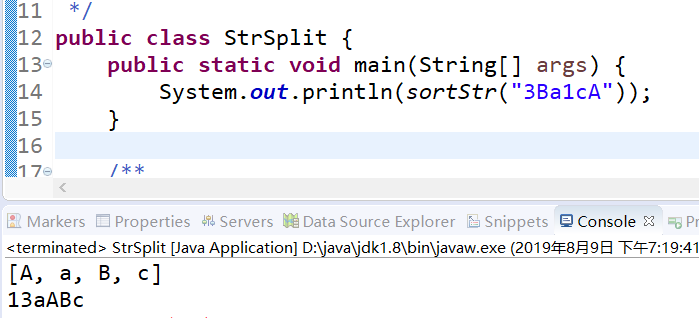• ## java字符串排序

千次阅读 2019-08-09 19:29:18
java字符串排序 今天收到一个公司的面试邀请，人还没去，直接面试题都发过来了，真仗义啊。哈哈哈，我看了一下面试题，难度还是挺高的，怪不得直接给我。 我大致看了一下关于java方面的面试题，我还是对其中一个编程...
java字符串排序
今天收到一个公司的面试邀请，人还没去，直接面试题都发过来了，真仗义啊。哈哈哈，我看了一下面试题，难度还是挺高的，怪不得直接给我。
我大致看了一下关于java方面的面试题，我还是对其中一个编程题挺感兴趣的，题目如下：就是对一个无序字符串进行排序，主要难度在怎么处理字母的排序，Arrays类和Collections类提供的排序方法都是大写字母在前的，题目要求的是小写在前。这就是个考点，废话不多说直接上代码：

import java.util.Arrays;
import java.util.Collections;
import java.util.List;

/**
*
* @author 谢辉 2019.8.9
*
*/
public class StrSplit {
public static void main(String[] args) {
System.out.println(sortStr("3Ba1cA"));
}

/**
* 思路：
* 1、先对字符串拆分，进行第一次排序，目的是把数字提取出来
* 2、数字提取出来后利用集合工具类得到排序后的字母（缺点是大写的字母在前，不是想要的结果）
* 3、利用冒泡排序改变大小写
*
* @param str
* @return
*/
public static String sortStr(String str) {
// 形参拆分
char[] charArray = str.toCharArray();
// 存取结果
StringBuilder result = new StringBuilder();
// 对数组排序：数字、大写字母、小写字母
Arrays.sort(charArray);

int index = 0;
// 查找最后一位数字紧挨着的大写字母下标（将会使用该下标截取字符串）
// 0的ASCII码是48 9的ASCII码是57
for (int i = 0; i < charArray.length; i++) {
if (Integer.valueOf(charArray[i]) > 57) {
index = i;
break;
}
}
// 先截取出所有数字
String temp = String.valueOf(charArray);
result.append(temp.substring(0, index));

// 剩下纯字母字符串
String newStr = temp.substring(index);
// 利用集合对字母数组排序
char[] array = newStr.toCharArray();
for (char c : array) {
}
Collections.sort(list, String.CASE_INSENSITIVE_ORDER);
System.out.println(list.toString());// [A, a, B, b, c, d, Z, z]

// 排序后的字母都是大写的在前面
String[] strTemp = new String[list.size()];
for (int i = 0; i < strTemp.length; i++) {
strTemp[i] = list.get(i);
}
// 利用冒泡排序进行对比，改变大小写
for (int i = 0; i < strTemp.length - 1; i++) {
for (int j = 0; j < strTemp.length - 1 - i; j++) {
if (strTemp[j].equals(strTemp[j + 1])) {
continue;
} else if (strTemp[j].equalsIgnoreCase(strTemp[j + 1])) {
strTemp[j] = strTemp[j].toLowerCase();
strTemp[j + 1] = strTemp[j + 1].toUpperCase();
}
}
}

// 拼接字符串
for (int i = 0; i < strTemp.length; i++) {
result.append(strTemp[i]);
}

return result.toString();
}
}有办法继续上代码：
import java.util.Arrays;

public class A {
public static void main(String[] args) {
System.out.println(sortStr("36z1ZaBbdc8","aA0"));
}

/**
*
* @param str 需要排序的字符串
* @param splitRule 定义的规则：默认是0Aa
* @return 排序后的字符串
* 规则介绍：
* 对于排序规则定义了一个0、A、a三个字符组成的字符串规则，
* 0aA表示数字所有排序后的数字在前面，所有排序后的小写字母在中间，所有大写字母在最后
* 规则有：0Aa(默认)、A0a、0aA、a0A、aA0
*/
public static String sortStr(String str, String splitRule) {
/**
* 思路：
* 1、把字符串拆开，用Arrays工具类进行排序，排序规则是：数字-大写字母-小写字母
* 2、根据规则按照ASCII码再次拆分字符串
* 3、第一次拆分是把数字部分拆出来
* 4、第二次把所有大写字母拆分出来
* 5、第三次把所有小写字母拆分出来
* 6、按要求再次拼接
*/
// 0的ASCII码是48 9的ASCII码是57
// A的ASCII码是65 Z的ASCII码是90
// a的ASCII码是97 z的ASCII码是122

// 形参拆分
char[] charArray = str.toCharArray();
// 存取结果
StringBuilder result = new StringBuilder();
// 对数组排序：数字、大写字母、小写字母
Arrays.sort(charArray);
if (null == splitRule || "".equals(splitRule)) {
return String.valueOf(charArray);
}

/*
* 开始第一次截取
*/
int index = 0;
// 查找最后一位数字紧挨着的大写字母下标（将会使用该下标截取字符串）
for (int i = 0; i < charArray.length; i++) {
if (Integer.valueOf(charArray[i]) > 57) {
index = i;
break;
}
}
// 第一次截取：所有数字
String temp = String.valueOf(charArray);
// result.append(temp.substring(0, index));

/*
* 开始第二次截取
*/
// 该字符串就是去掉数字的字符串了
String newStr = temp.substring(index);
int index2 = 0;
char[] newCharArr = newStr.toCharArray();
// 找到最后一个大写字母紧挨着的小写字母下标(使用该下标截取字符串)
for (int i = 0; i < newCharArr.length; i++) {
if (Integer.valueOf(newCharArr[i]) > 90) {
index2 = i;
break;
}
}
// 截取到的就是所有小写字母
// result.append(newStr.substring(index2));

/*
* 开始第三次截取
*/
// 截取到的就是所有大写字母
// result.append(newStr.substring(0, index2));

String finalResult = "";
// 为了程序的扩展性，对一下排序规则做了定义，就是按照规则追加字符串
if ("0aA".equals(splitRule)) {
result.append(temp.substring(0, index));
result.append(newStr.substring(index2));
result.append(newStr.substring(0, index2));
finalResult = result.toString();
} else if ("A0a".equals(splitRule)) {
result.append(newStr.substring(0, index2));
result.append(temp.substring(0, index));
result.append(newStr.substring(index2));
finalResult = result.toString();
} else if ("aA0".equals(splitRule)) {
result.append(newStr.substring(index2));
result.append(newStr.substring(0, index2));
result.append(temp.substring(0, index));
finalResult = result.toString();
}else if ("a0A".equals(splitRule)) {
result.append(newStr.substring(index2));
result.append(temp.substring(0, index));
result.append(newStr.substring(0, index2));
finalResult = result.toString();
}
return finalResult;

}
}

总之面试官的要求千变万化，解决问题先有思路，然后问题迎刃而解…
在这里只是分享面试题，希望技术大牛勿喷，谢谢大牛的照顾。同时希望小伙伴们指出错误和不足之处，谢谢大家！


展开全文String
• import java.util.Arrays; /** * Created by IntelliJ IDEA. * User: 蔡燃 * Date: 2019/9/19 * explain: */ public class Test { public static void main(String[] args) { String str="qwevzxca...
import java.util.Arrays;

/**
* Created by IntelliJ IDEA.
* User: 蔡燃
* Date: 2019/9/19
* explain:
*/
public class Test {
public static void main(String[] args) {
String str="qwevzxcaas差睡吧睡吧大";
char[] strChar=str.toCharArray();
Arrays.sort(strChar);
System.out.println(String.valueOf(strChar));

}
}


展开全文• ## Java字符串排序

千次阅读 2019-02-03 20:56:14
编写一个程序，将输入字符串中的字符按如下规则排序。 规则 1 ：英文字母从 A 到 Z 排列，不区分大小写。 如，输入： Type 输出： epTy 规则 2 ：同一个英文字母的大小写同时存在时，按照输入顺序排列。 如，输入： ...
题目描述：
编写一个程序，将输入字符串中的字符按如下规则排序。
规则 1 ：英文字母从 A 到 Z 排列，不区分大小写。
如，输入： Type   输出： epTy
规则 2 ：同一个英文字母的大小写同时存在时，按照输入顺序排列。
如，输入： BabA   输出： aABb
规则 3 ：非英文字母的其它字符保持原来的位置。
如，输入： By?e   输出： Be?y
例：
输入：
输出：
A aaAAbc dFgghh: iimM nNn oooos Sttuuuy (2012/8).
代码：
import java.util.Scanner;

public class Test {
public static void main(String[] args) {
Scanner scanner = new Scanner(System.in);
String sc = scanner.nextLine();
String[] a = new String[sc.length()];
String[] b = new String[sc.length()];
for (int i = 0; i < sc.length(); i++) {//把字符串存在数组中
a[i] = sc.substring(i, i + 1);
}
int count = 0;
for (int i = 0; i < sc.length(); i++) {
if (97 <= a[i].charAt(0) && a[i].charAt(0) <= 122 ||
65 <= a[i].charAt(0) && a[i].charAt(0) <= 90){
b[count++] = "没";
}else {
b[count++] = a[i];
}
}
count = 0;
char n = 'a';
char m = 'A';
int tmp = 26;//26个字母
while (tmp-- > 0) {
for (int i = 0; i < sc.length(); i++) {
if (97 <= a[i].charAt(0) && a[i].charAt(0) <= 122 ||
65 <= a[i].charAt(0) && a[i].charAt(0) <= 90) {//判断是否是字母
if (a[i].charAt(0) == n  || a[i].charAt(0) == m ) {//是否是需要的字母
for (int j = 0;j<sc.length();j++){
if (b[j] == "没"){
b[j] = a[i];
break;
}
}
}
}
}
n += 1;
m += 1;
}
for (int i = 0; i < sc.length(); i++) {
System.out.print(b[i]);
}
}
}



展开全文• JAVA字符串排序去重 场景问题：在DB中我们可能会对数据的ID进行拼接，有时会存在部分ID相同的情况。此时需要对查询出来的这个拼接数据（type：String）进行去重。 实现：此处采用JAVA8的Stream()操作进行实现，做...

场景问题：在DB中我们可能会对数据的ID进行拼接，有时会存在部分ID相同的情况。此时需要对查询出来的这个拼接数据（type：String）进行去重。

实现：此处采用JAVA8的Stream()操作进行实现，做个记录，方便下次使用。

CODE

在本业务中拼接的ID数据一般使用",“和”_"作为拼接符，所以code中的delimiter是写死的两种情况，后续待优化。

去重数据：

String value1 = “aa,bb,cc,aa,dd,ee,aa,”
String value2 = “12_15_30_12_100_1_58_90_210”
》执行结果如下：
1） “aa,bb,cc,dd,ee”
2） “1_12_15_30_58_90_100_210”


private String removeDuplicateData(String value) {
//工具类判断字符串非空
if (CommonUtil.isEmptyString(value)) {
retun null;
}
String delimiter = value.contains("_") ? "_":",";
String lastCharacter = value.substring(value.length()-1);
if (lastCharacter.equals(delimiter)) {
value = value.substring(0,value.length()-1);
}
if (value.equals(delimiter)) {
return null;
}
List<String> targetList = Arrays.asList(value.split(delimiter));
//JAVA8的stream()操作,sorted()用来排序，distinct()用来去重
return targetList.stream().sorted(Comparator.comparing(String::hashCode)).distinct().collect(Collectors.joining(delimiter));
}

这个方法由于在业务中侧重对数字字符串进行去重排序，字符的排序建议自己测试通过后再进行使用。


展开全文• Java字符串排序设计（升序排列） package 六月; import java.util.Arrays; /* * 字符串排序设计（升序排列） * 20 78 9 -7 88 36 29 */ public class Wrapper { public static void main(String[] args) { ...
•string
• //Java 字符串如何排序比较 // 1、如果字符串相等返回值0 // 2、如果第一个字符和参数的第一个字符不等,结束比较,返回他们之间的差值（ascii码值）（负值前字符串的值小于后字符串，正值前字符串大于后字符串...
• 示例,将字符串"91 27 46 38 50" 转换成"27 38 46 50 91" 后输出. public class RegexDemo { public static void main(String[] args) { String s = "91 27 46 38 50"; //把字符串...
• 解决思路： 在Java中，排序需要复写的是 equals 方法 和 Comparable 接口 的public int compareTo(T o); 方法 步骤： 1. 使用正则表达式来判断数字，多个连续的数字作为一组, 2. 一次检索出数字组合， 3. 检出下...正则表达式 数字 Windows
• 图片名命名规则: sku-图片数字序号.jpg 或 特殊字符-图片数字序号.jpg 或 中文.图片数字序号.jpg 等等 命名规则很乱,因为获取的是第三方的数据,但是不变的是图片数字序号前面的前缀,不同的sku中间的分隔符可能也会变...
• 请对一组字符串进行排序字符串由大小写字母和数字组成，需要满足一下比较规则 长度不同时，长度较短在排前面。 长度相同时，按照字典顺序排列（AaBb-Zz，0-9顺序），即大写字母在小写字母前，数字排在字母后。 ...
• import java.io.*; import java.util.*; public class Main { public static void main(String args[]) throws Exception{ Scanner cin = new Scanner(System.in); String str ; int n;string exception import class
• Java字符串排序 文章目录Java字符串排序排序方法概述键索引计数法低位优先的字符串排序（LSD）高位优先的字符串排序（MSD）三向字符串快速排序 排序方法概述 对于许多应用，决定顺序的键都是字符串。本篇讲述如何...
• java:字符串排序 题目 问题描述 　输入一个小写字符串，按从小到大的顺序输出。 输入格式 　bcaed 输出格式 　abcde 　顶格输出，中间没有空格 样例输入 一个满足题目要求的输入范例。 例： bcaed 样例输出 与上面的...
• 题目：字符串排序。 import java.util.*; public class test{ public static void main(String[] args) { ArrayList<String> list=new ArrayList<String>(); list.add("010101"); list.add("010003...算法
• Java环境下，对字符串数组或者对字符串排序都是一件很容易的
• ## Java实现字符串排序

千次阅读 2020-06-09 01:31:44
import java.util.Arrays; import java.util.Scanner; public class StrSort { public static void main(String[] args) { Scanner sc = new Scanner(System.in);//引入输入的功能 String totalStr = "";//因不...
• ## java实现字符串排序

千次阅读 2014-11-23 17:10:17
有一些A、C、M组成的字符串，将其按字符A排序 输入：一组测试数据，输入数据由若干行组成，每行是字符A、C或M组成的字符串。(每个字符串长度不超过1200)正则表达式 string
• java字符串转字符串数组Java String array is used to hold fixed number of Strings. String array is very common in simple java programs, specially among beginners to java and to test some specific ...算法 python 编程语言
• ## Java_字符串排序

千次阅读 2019-08-06 20:14:10
//字符串排序. public class Test { public static void main ( String [ ] args ) { String [ ] strs = { "dog" , "cat" , "apple" , "app" , "APP" , "application" , "ball" } ; ...算法
• 字符串排序   用Java编写一个能对一组字符串按字典序升序排序的程序 输入为N和N行字符串，需要按行输出字符串升序排序的结果 如输入 3 Abc Abe Abd 输出： Abc Abd Abe import java.io.*; import java.util.*; ...
• ## java中的字符串排序

万次阅读 2018-03-06 23:04:11
要求：对 "abc","...字段进行排序，要求满足其为升序排列同时每个字符串里也是升序排列 public class Test { public static void main(String[] args) { String[] str = new String
• ## Java日期字符串排序

千次阅读 2016-12-01 15:35:08
一、示例代码class test { public static void main (String[] args) throws java.lang.Exception { TreeSet<String> dates = new TreeSet(); dates.add("2016-10-20"); dates.a...

# java字符串排序java 订阅